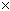Question from Randy: Calculate the amount(in gallons)of rainfall .98 inches will put over an area of 3.76 acres. Thank you for your assistance. Hi Randy. You have a depth measurement (0.98 inches) and an area measurement (3.76 acres) and want a volume measurement (in gallons). Recall that to get volume, you can multiply the area by the depth. So the answer is volume = areadepth, but you will have to sort out your units first. We may as well do everything in inches, inches2 and inches3 , so we have to convert 3.76 acres into square inches. According to my reference material, an acre is 4840 yards2 . Since there are 36 inches in a yard, we can substitute that in: 4840 yards2 = 4840 (36 inches)2 = 4840362 inches2 . If we multiply by the depth of 0.98 inches, we get this: (0.98 inches)(4840362 inches2) = 0.984840362 inches3 (I'll let you do the actual calculation). This gives you the volume of rain in cubic inches, but you want it in gallons. There are different gallon sizes in different parts of the world. Many parts of the world, such as the UK, use gallons that are equivalent to 277.4 inches3 . Americans use a smaller gallon, which is 231 inches3 . So you have to divide your volume in cubic inches by this conversion quantity to get gallons. Here's a plug for metric. The same question, using metric units would ask something like this: Calculate the amount of rainfall in liters that fall to a depth of 3 cm over 1.5 hectares. Metric uses base 10, so things are generally easier, because you just multiply and divide by powers of ten. Each hectare is 10 000 meters2 . Each centimeter is 0.01 meters. That means the volume in meters3 is: (3.01 meters)(1.5 x 10 000 meters2 ) = 4 500 meters3 . And since there are 1000 liters in a meter3 , the answer would be 4 500 000 liters (also called 4.5 megaliters). Hope this helps, Stephen La Rocque.